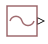# PS Sine Wave

Generate sine wave physical signal, using simulation time as time source

Since R2021a

•Libraries:
Simscape / Foundation Library / Physical Signals / Sources

## Description

The PS Sine Wave block outputs a sinusoidal waveform, while providing flexibility in how you specify the sine wave frequency.

In the SI unit system, the unit of frequency is hertz (`Hz`), which is defined as `1/s`. However, you can also use angular velocity units, such as `rad/s`, `deg/s`, and `rpm`, to measure frequency for cyclical processes. This approach is consistent with frequency defined as revolutions per second in a mechanical context, or cycles per second in an electrical context, and lets you write frequency-dependent equations without requiring the `2*pi` conversion factor. For more information, see Units for Angular Velocity and Frequency.

The Frequency specification parameter lets you choose the method of specifying the frequency of the sine wave:

• If you select `Frequency(SI)`, specify the frequency in `Hz` or in units directly convertible to Hz (such as `kHz`, `MHz`, and `GHz`). The underlying block equation is then:

`$O=amplitude\cdot \mathrm{sin}\left(2\pi \cdot frequency_si\cdot time+phase\right)+bias$`
• If you select `Angular`, specify angular frequency in `rad/s`, `deg/s`, or `rpm`. The underlying block equation is then:

`$O=amplitude\cdot \mathrm{sin}\left(angular_frequency\cdot time+phase\right)+bias$`

where

 O Physical signal at the output port amplitude Amplitude parameter value frequency_si Frequency (SI) parameter value angular_frequency Angular frequency parameter value time Simulation time phase Phase parameter value bias Bias parameter value

The Amplitude and Bias parameter values determine whether the output signal is a scalar, vector, or matrix. If one of these parameters is a vector or a matrix, the other must be a vector or a matrix of the same size or a scalar. The output signal is then also a vector or a matrix of the same size.

Amplitude and Bias must have commensurate units. The unit at the output port is determined by unit propagation rules. If the two parameters have the same units, then the output signal unit is also the same. If the parameter units are different, then the unit of the output signal is the base unit commensurate with the units of the parameters. For more information, see Physical Signal Unit Propagation.

## Ports

### Output

expand all

Output physical signal. The signal size matches the size of the Amplitude and Bias parameter values. The signal unit is determined by the Amplitude and Bias parameter units.

The port name is not visible in the block icon, but you can see this name in the underlying source file by clicking the Source code link in the Description tab of the block dialog box.

## Parameters

expand all

The amplitude of the sinusoidal waveform. The first edit box represents the parameter value. You can specify a scalar, vector, or matrix. By default, the value is a scalar, `1`. If you specify a vector or a matrix, Bias must also be a vector or a matrix of the same size or a scalar.

The second combo box represents the parameter unit, which also determines the unit of the output signal. By default, the unit is `1` (unitless). You can select a unit from the drop-down list or type the desired unit name, such as `Pa`, or a valid expression, such as `m^2`. For more information and a list of unit abbreviations, see How to Specify Units in Block Dialogs and Unit Definitions.

Bias of the sinusoidal waveform, that is, the constant value added to the sine wave to produce the output. The first edit box represents the parameter value. You can specify a scalar, vector, or matrix. By default, the value is a scalar, `0`. If you specify a vector or a matrix, Amplitude must also be a vector or a matrix of the same size or a scalar.

The second combo box represents the parameter unit. The specified unit must be commensurate with the unit of the Amplitude parameter. By default, the unit is `1` (unitless). You can select a unit from the drop-down list or type the desired unit name, such as `Pa`, or a valid expression, such as `m^2`. For more information and a list of unit abbreviations, see How to Specify Units in Block Dialogs and Unit Definitions.

Select whether to specify frequency in SI system units, such as Hz, or angular units, such as rad/s. The underlying block equation changes based on your selection because angular units do not require the `2*pi` conversion factor.

Frequency of the sine wave, specified in SI frequency units, such as `Hz`, `kHz`, `MHz`, or `GHz`.

#### Dependencies

To enable this parameter, set Frequency specification to `Frequency (SI)`.

Frequency of the sine wave, specified in angular frequency units, such as `rad/s`, `deg/s`, or `rpm`.

#### Dependencies

To enable this parameter, set Frequency specification to `Angular`.

Phase shift of the sine wave.

## Version History

Introduced in R2021a